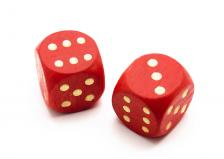# How to Solve the Famous Birthday Problem

Jason Marshall in QuickandDirtyTips:As you may know, the quadrennial World Cup was recently played in Brazil. As you may not know, each of the 32 teams in this year's tournament had 23 players on their roster. This week's Math Dude episode has absolutely nothing to do with soccer or the World Cup, so why have I mentioned this fun fact? Allow me to answer this question with a question: What are the odds that two players from one World Cup team share a birthday? It seems like the chances are pretty slim. After all, there are 365 days in a year, and only 23 players on a team—so surely there's not much chance of a shared birthday, right? Believe it or not, wrong. As we'll soon find out, there's actually a decent chance that many—perhaps even the majority—of World Cup teams will have a pair of players who share a birthday. How is that possible? Keep on reading to find out!

To help us understand the probabilities of shared birthdays, let's think a bit about the probabilities of rolling dice. To begin with, what's the probability of tossing 6 when you roll a single die? Since dice have 6 sides, the probability must be 1 out of 6, or 1/6. Now let's imagine that we roll the same die twice. What's the probability that we get the same number—no matter what it is—twice? Whatever we get on the first roll, there's a 1 out of 6 chance that we get the same number again. So, once again, the probability is 1/6. If we roll the same die three times, what's the probability of getting the same number three times in a row? As we've seen, there's a 1/6 chance that the first two rolls will give the same number. And whatever that number is, there's a 1/6 chance that the third roll will yield it again. So the probability of rolling the same number three times in a row is 1/6 x 1/6 = 1/36.

More here.